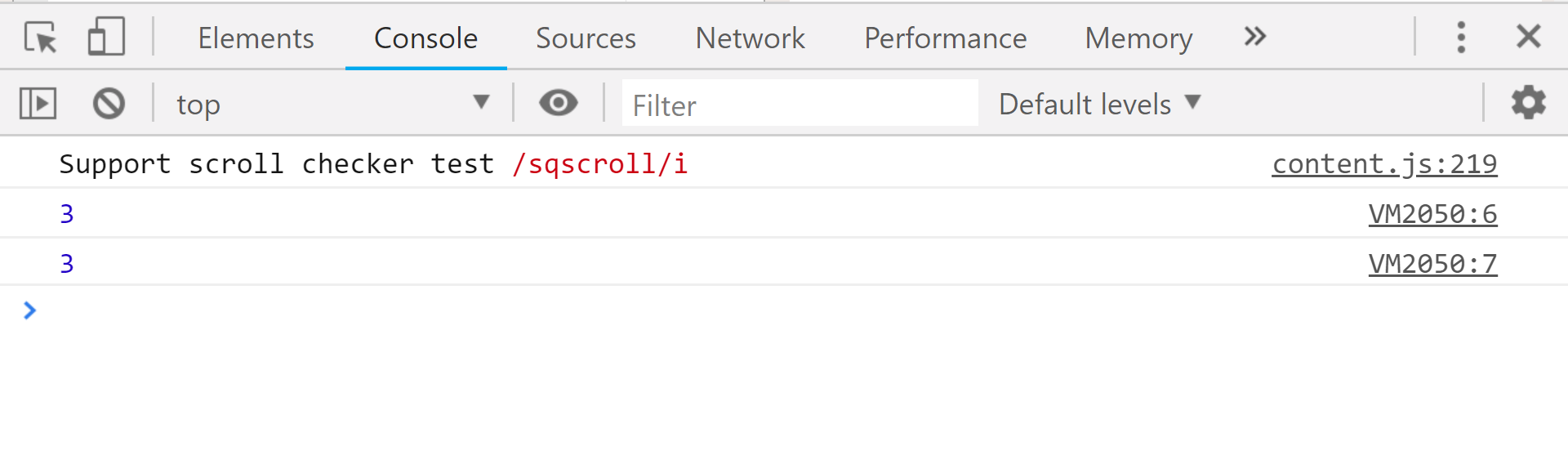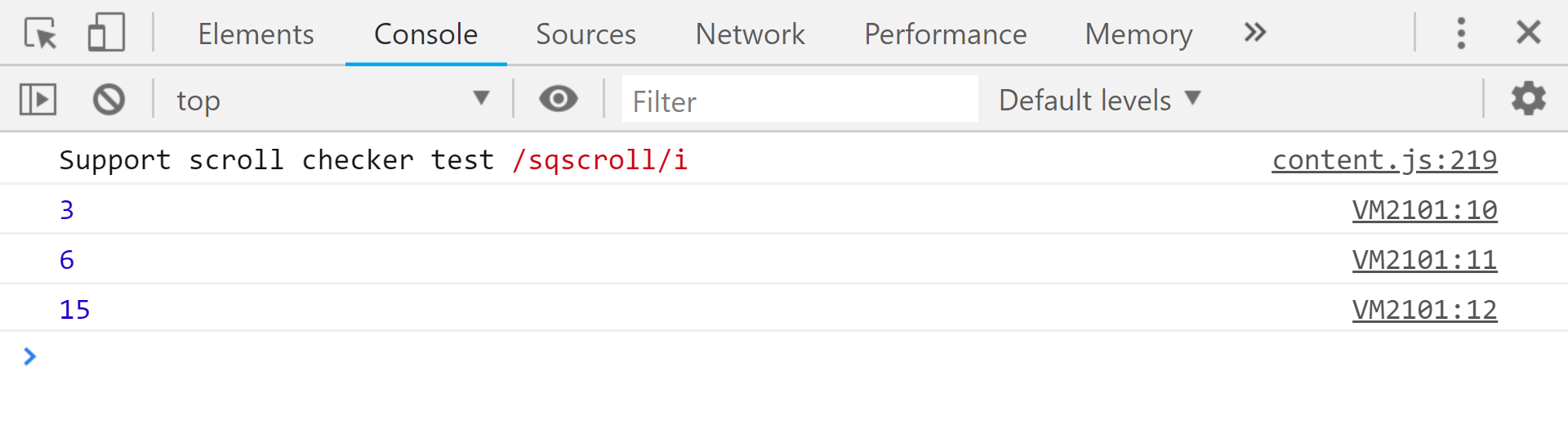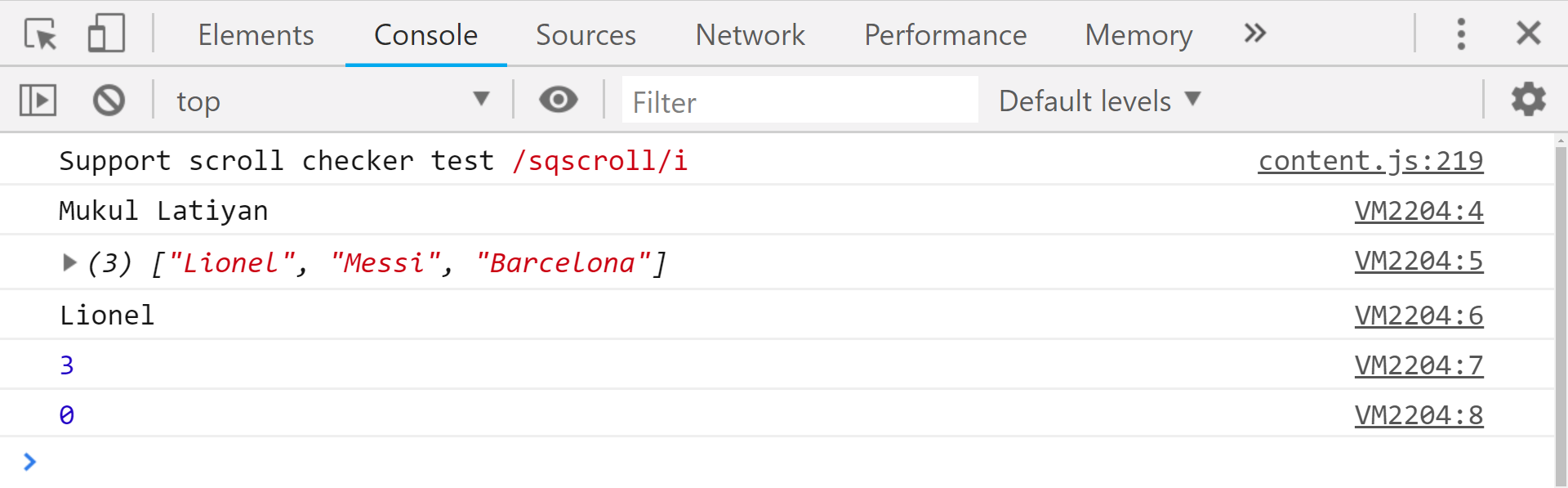Related Articles
JavaScript | Rest parameter
• Last Updated : 16 Apr, 2019

Rest parameter is an improved way to handle function parameter, allowing us to more easily handle various input as parameters in a function. The rest parameter syntax allows us to represent an indefinite number of arguments as an array. With the help of a rest parameter a function can be called with any number of arguments, no matter how it was defined. Rest parameter is added in ES2015 or ES6 which improved the ability to handle parameter.

Note: In order to run the code in this article make use of the console provided by the browser or use an online tool like repl.it.

Syntax:

```function functionname[...parameters]//... is the rest parameter
{
statement;
}```

Note: When … is at the end of function parameter, it is the rest parameter. It stores n number of parameters as an array. Let’s see how the rest parameter works:

 `// Without rest parameter ` `function` `fun(a, b){ ` `    ``return` `a + b; ` `} ` `console.log(fun(1, 2)); ``// 3 ` `console.log(fun(1, 2, 3, 4, 5)); ``// 3                 `

Output:In the above code, no error will be thrown even when we are passing arguments more than the parameters, but only the first two arguments will be evaluated. It’s different in the case with rest parameter. With the use of the rest parameter, we can gather any number of arguments into an array and do what we want with them.
Javascript code demonstrating addition of numbers using rest parameter.

 `// es6 rest parameter ` `function` `fun(...input){ ` `    ``let sum = 0; ` `    ``for``(let i of input){ ` `        ``sum+=i; ` `    ``} ` `    ``return` `sum; ` `} ` `console.log(fun(1,2)); ``//3 ` `console.log(fun(1,2,3)); ``//6 ` `console.log(fun(1,2,3,4,5)); ``//15                  `

Output:We were able to get the sum of all the elements that we enter in the argument when we call the fun() function. We get the sum of all the elements in the array by making use of the for..of loop which is used to traverse the iterable elements inside an array.
Note: The rest parameter have to be the last argument, as its job is to collect all the remaining arguments into an array. So having a function definition like the code below doesn’t make any sense and will throw an error.

 `// non-sense code ` `function` `fun(a,...b,c){ ` `     ``//code ` `     ``return``; ` `} `

Let’s make use of the rest parameter with some other arguments inside a function,like this:

 `// rest with function and other arguments ` `function` `fun(a,b,...c){ ` `    ``console.log(`\${a} \${b}`); ``//Mukul Latiyan ` `    ``console.log(c);  ``//[ 'Lionel', 'Messi', 'Barcelona' ] ` `    ``console.log(c); ``//Lionel ` `    ``console.log(c.length); ``//3 ` `    ``console.log(c.indexOf(``'Lionel'``)); ``//0 ` `} ` `fun(``'Mukul'``,``'Latiyan'``,``'Lionel'``,``'Messi'``,``'Barcelona'``); `

Output:In the above code sample, we passed the rest parameter as the third parameter and then we basically called the function fun() with five arguments and the first two were treated normally and the rest were all collected by the rest parameter and hence we get ‘Lionel’ when we tried to access c and it is also important to note that the rest parameter gives an array in return and we can make use of the array methods that the javascript provides us.My Personal Notes arrow_drop_up
Recommended Articles
Page :